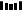## 概述

TwoSum 作为 LeetCode 的第一题存在，想必大家应该对其并不陌生。如果仅仅是看这道题目本身，并不难，思想也特别的简单。

## 两种思路

### 1、Hash 表的做法

public int[] twoSum(int[] nums, int target) {    Map<Integer, Integer> remainValues = new HashMap<>();    for (int i = 0; i < nums.length; ++i) {        if (remainValues.containsKey(target - nums[i])) {            int anotherIndex = remainValues.get(target - nums[i]);            return new int[] {i, anotherIndex};        }        remainValues.put(nums[i], i);    }    return new int[] {-1, -1};}

• 由于使用了 Hash 表，空间复杂度是 O(n) 的，另外就是最差的情况，数组中的每个元素都要遍历到，因此时间复杂度也是 O(n)

• 从时间上面来看，这个算法肯定是最优的，这很好理解，你要在数组中寻找配对的答案，数组当中的数肯定都需要看一遍。

### 2、排序加双指针的思路

public int[] twoSum(int[] n, int t) {    if (n == null || n.length == 0) {        return new int;    }    Arrays.sort(n);    int[] result = new int;    int l = 0, r = n.length - 1;    while (l < r) {        if (n[l] + n[r] == t) {            result = n[l];            result = n[r];            return result;        } else if (n[l] + n[r] < t) {            l++;        } else {            r--;        }    }    return new int;}

• 每次判断的时候，我们将左右两个指针指向的元素加起来的和与我们要找的 target 对比，如果比 target 小，也就是说明如果在左指针不变的情况下，左指针加上左右指针中间的任意一个元素都会比 target 小，这也说明左指针不可能是我们要找的答案，因此向右移动左指针。

• 如果是加起来和我们要找的 target 对比，比 target 大，分析类似，这时需要将右指针向左移动。

• 如果题目要求输出所有可能的答案，该怎么处理？

• 如果题目要求输出所有可能的答案，并且数组中有重复元素该怎么处理？

• 如果题目要求找到比 target 小/大 的配对该怎么处理？

• 如果要找出两数之差等于 target 的配对，该如何进行？

• TwoSum 的解题思路是否可以拓展到 TreeSum 或者更多的配对？

## 变形题目分析

### 题目解析

• 对于 Hash 表的方法来说，其实你并不清楚之前是否添加过相同的答案，因此我们考虑使用一个集合去存储答案，保证答案的不重复性；

• 对于排序的方法来说，去重的方式有所不同，排完序后，相同的元素会挨在一起，对于之前考虑过的元素，我们只需要略过就行，指针的移动很好地保证了这一点。

### 代码实现

public List<int[]> twoSum6(int[] nums, int target) {    if (nums == null || nums.length < 2)        return 0;    Arrays.sort(nums);    List<int[]> results = new ArrayList<>();    int left = 0, right = nums.length - 1;    while (left < right) {        int v = nums[left] + nums[right];        if (v == target) {            int[] result = {nums[left], nums[right]};            results.add(result);            left++; right--;            while (left < right && nums[right] == nums[right + 1]) {                right--;            }            while (left < right && nums[left] == nums[left - 1]){                left++;            }        } else if (v > target) {            right--;        } else {            left++;        }    }    return results;}

### 题目描述

输入：A = [34,23,1,24,75,33,54,8], K = 60输出：58解释：34 和 24 相加得到 58，58 小于 60，满足题意。

输入：A = [10,20,30], K = 15输出：-1解释：我们无法找到和小于 15 的两个元素。

1. 1 &lt;= A.length &lt;= 100

2. 1 &lt;= A[i] &lt;= 1000

3. 1 &lt;= K &lt;= 2000

### 代码实现

public int twoSumLessThanK(int[] A, int K) {    if (A == null || A.length == 0) {        return -1;    }    Arrays.sort(A);    int l = 0, r = A.length - 1;    int result = Integer.MIN_VALUE;    while (l < r) {        if (A[l] + A[r] >= K) {            r--;        } else {            result = Math.max(result, A[l] + A[r]);            l++;        }    }    return result == Integer.MIN_VALUE ? -1 : result;}

### 题目描述

add 操作 –  对内部数据结构增加一个数。
find 操作 – 寻找内部数据结构中是否存在一对整数，使得两数之和与给定的数相等。

add(1); add(3); add(5);find(4) -> truefind(7) -> false

add(3); add(1); add(2);find(3) -> truefind(6) -> false

### 代码实现

private Map<Integer, Integer> elements;private int MAX_VALUE = Integer.MIN_VALUE;private int MIN_VALUE = Integer.MAX_VALUE;/** Initialize your data structure here. */public TwoSum() {    elements = new HashMap<>();}/** Add the number to an internal data structure.. */public void add(int number) {    elements.put(number, elements.getOrDefault(number, 0) + 1);    MAX_VALUE = Math.max(MAX_VALUE, number);    MIN_VALUE = Math.min(MIN_VALUE, number);}/** Find if there exists any pair of numbers which sum is equal to the value. */public boolean find(int value) {    if (value < 2 * MIN_VALUE || value > 2 * MAX_VALUE) {        return false;    }    for (int i : elements.keySet()) {        if (i * 2 == value && elements.get(i) >= 2) {            return true;        } else if (i * 2 != value && elements.containsKey(value - i)) {            return true;        }    }    return false;}

### 题目描述

例如, 给定数组 nums = [-1, 0, 1, 2, -1, -4]，满足要求的三元组集合为：[  [-1, 0, 1],  [-1, -1, 2]]

### 代码实现

// sort + two pointerspublic List<List<Integer>> threeSum(int[] nums) {    if (nums == null || nums.length < 3) {        return new ArrayList<>();    }    Arrays.sort(nums);    List<List<Integer>> results = new ArrayList<>();    for (int i = 0; i < nums.length - 2; ++i) {        if ((i != 0) && (nums[i] == nums[i - 1])) {            continue;        }        List<Integer> result = new ArrayList<>();        twoSum(results, nums, i + 1, -nums[i]);        result = null;    }    return results;}private void twoSum(List<List<Integer>> results,                    int[] nums,                    int startIndex,                    int target) {            int left = startIndex, right = nums.length - 1;    while (left < right) {        if (nums[left] + nums[right] > target) {            right--;        } else if (nums[left] + nums[right] < target) {            left++;        } else {            List<Integer> result = new ArrayList<>();            result.add(-target);            result.add(nums[left]);            result.add(nums[right]);            results.add(result);            left++; right--;            while ((left < right) && (nums[left - 1] == nums[left])) {                left++;            }            while ((right > left) && (nums[right + 1] == nums[right])) {                right--;            }        }    }}

// HashSetpublic List<List<Integer>> threeSum(int[] nums) {    if (nums == null || nums.length < 3) {        return new ArrayList<>();    }    Arrays.sort(nums);    Set<List<Integer>> resultsSet = new HashSet<>();    List<List<Integer>> results = new ArrayList<>();    for (int i = 0; i < nums.length - 2; ++i) {        if ((i != 0) && (nums[i - 1] == nums[i])) {            continue;        }        Set<Integer> existedValue = new HashSet<>();        for (int j = i + 1; j < nums.length; ++j) {            if (!existedValue.contains(nums[j])) {                existedValue.add(-nums[j] - nums[i]);            } else {                List<Integer> result = new ArrayList<>();                Collections.addAll(result, nums[i], -nums[j] - nums[i], nums[j]);                resultsSet.add(result);            }        }        existedValue = null;    }    results.addAll(resultsSet);    return results;}

### 题目描述

输入: [2,2,3,4]输出: 3解释:有效的组合是: 2,3,4 (使用第一个 2)2,3,4 (使用第二个 2)2,2,3

• 数组长度不超过1000。

• 数组里整数的范围为 [0, 1000]。

### 题目解析

a < b < c && a + b > c => 三角形

### 代码实现

public int triangleCount(int[] S) {    if (S == null || S.length == 0) {        return 0;    }    Arrays.sort(S);    int result = 0;    for (int i = S.length - 1; i >= 2; --i) {        int l = 0, r = i - 1;        while (l < r) {            if (S[i] < S[l] + S[r]) {  // S[i] < S[l] + S[r] && S[i] > S[r] > S[l]                result += r - l;  //  直接加上可能的个数                r--;            } else {                l++;            }        }    }    return result;}

### 题目描述

给定数组 nums = [1, 0, -1, 0, -2, 2]，和 target = 0。满足要求的四元组集合为：[  [-1,  0, 0, 1],  [-2, -1, 1, 2],  [-2,  0, 0, 2]]

### 题目分析

4Sum 的思路和 3Sum 的思路是一样的，只不过这时我们需要先将其转换成 3Sum 来处理，其实就是比 3Sum 多了一次选择。

### 代码实现

public List<List<Integer>> fourSum(int[] nums, int target) {    if (nums.length < 4) {        return new ArrayList<>();    }    Arrays.sort(nums);    List<List<Integer>> results = new ArrayList<>();    for (int i = 0; i < nums.length - 3; ++i) {        if (i != 0 && nums[i] == nums[i - 1]) {            continue;        }        List<List<Integer>> subResults = threeSum(nums, i + 1, target - nums[i]);        for (List<Integer> res : subResults) {            res.add(nums[i]);            results.add(res);        }    }    return results;}private List<List<Integer>> threeSum(int[] nums, int start, int target) {    List<List<Integer>> results = new ArrayList<>();    for (int i = start; i < nums.length - 2; ++i) {        if (i != start && nums[i] == nums[i - 1]) {            continue;        }        List<List<Integer>> res = twoSum(nums, i + 1, target - nums[i]);        for (List<Integer> r : res) {            r.add(nums[i]);            results.add(r);        }    }    return results;}private List<List<Integer>> twoSum(int[] nums, int start, int target) {    List<List<Integer>> results = new ArrayList<>();    int l = start, r = nums.length - 1;    while (l < r) {        if (nums[l] + nums[r] < target) {            l++;        } else if (nums[l] + nums[r] > target) {            r--;        } else {            List<Integer> result = new ArrayList<>();            result.add(nums[l]); result.add(nums[r]);            results.add(result);            r--; l++;            while (l < r && nums[r] == nums[r - 1]) {                r--;            }            while (l < r && nums[l] == nums[l + 1]) {                l++;            }        }    }    return results;}

### 题目解析

num1 + num2 = target

num1 - num2 = target or num2 - num1 = target

### 代码实现

public int[] twoDiff(int[] n, int t) {    if (n == null || n.length == 0) {        return new int;    }    Arrays.sort(n);    int[] result = new int;    int l = 0, r = 1;    while (r < n.length) {        if (l == r) {            r++;        }        if (n[r] - n[l] == t) {            result = n[l];            result = n[r];            return result;        } else if (n[r] - n[l] < t) {            r++;        } else {            l++;        }    }    return new int;}

## 总结

TwoSum 相关的问题就分析到这里，非常有趣的一点是，TwoSum 不仅可以扩展成 3Sum，4Sum，它也可以扩展为 KSum， 但是这里就需要用到动态规划的知识了，暂不在这里讨论。希望这次分享的内容对你有所帮助。1.程序员

2.【GitHub

3.【算法动画：七分钟理解什么是KMP算法

4.【数据结构十大经典排序算法动画与解析，看我就够了！